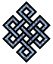fail2ban bad ip database: ip 217.128.2.139

| ip database | live view | stats | report | help | api key:

 ip: 217.128.2.139 hostname: LAubervilliers-656-1-203-139.w217-128.abo.wanadoo.fr country:[FR] France first reported: 03.08.2018 04:46.12 GMT+0200 last reported: 13.12.2018 00:50.20 GMT+0200 time period: 131d 21h 04m 08s total reports: 12 reported by: 3 host(s) filter(s): ssh (11) ssh (1) tor exit node no badips.com db Lookupport scan of '217.128.2.139':

[-hide]
```# Nmap 6.40 scan initiated Fri Aug  3 04:47:02 2018 as: /usr/bin/nmap -sU -sS -O 217.128.2.139
Warning: 217.128.2.139 giving up on port because retransmission cap hit (10).
Nmap scan report for LAubervilliers-656-1-203-139.w217-128.abo.wanadoo.fr (217.128.2.139)
Host is up (0.038s latency).
Not shown: 1993 filtered ports
PORT      STATE         SERVICE
21/tcp    closed        ftp
22/tcp    open          ssh
539/udp   open|filtered apertus-ldp
1027/udp  open|filtered unknown
18830/udp open|filtered unknown
58178/udp open|filtered unknown
58419/udp open|filtered unknown
No exact OS matches for host (If you know what OS is running on it, see http://nmap.org/submit/ ).
TCP/IP fingerprint:
OS:SCAN(V=6.40%E=4%D=8/3%OT=22%CT=21%CU=%PV=N%G=Y%TM=5B63CDAC%P=x86_64-pc-l
OS:inux-gnu)SEQ(SP=107%GCD=1%ISR=109%TI=Z%CI=I%TS=8)OPS(O1=M588ST11NW7%O2=M
OS:588ST11NW7%O3=M588NNT11NW7%O4=M588ST11NW7%O5=M588ST11NW7%O6=M588ST11)WIN
OS:(W1=7120%W2=7120%W3=7120%W4=7120%W5=7120%W6=7120)ECN(R=Y%DF=Y%TG=40%W=72
OS:10%O=M588NNSNW7%CC=Y%Q=)T1(R=Y%DF=Y%TG=40%S=O%A=S+%F=AS%RD=0%Q=)T2(R=N)T
OS:3(R=N)T4(R=Y%DF=Y%TG=40%W=0%S=A%A=Z%F=R%O=%RD=0%Q=)T5(R=Y%DF=Y%TG=40%W=0
OS:%S=Z%A=S+%F=AR%O=%RD=0%Q=)T6(R=Y%DF=Y%TG=40%W=0%S=A%A=Z%F=R%O=%RD=0%Q=)T
OS:7(R=N)U1(R=N)IE(R=N)

OS detection performed. Please report any incorrect results at http://nmap.org/submit/ .
# Nmap done at Fri Aug  3 05:36:12 2018 -- 1 IP address (1 host up) scanned in 2950.73 seconds
```
```Σ = 54 | Δt = 0.0049271583557129s
```